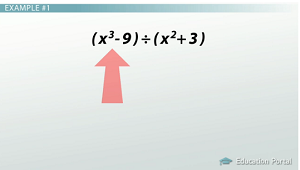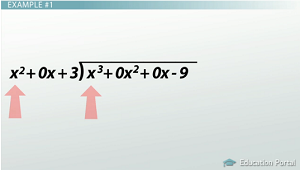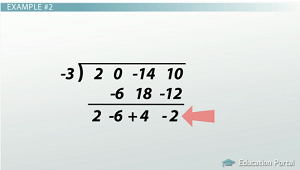# Dividing Polynomials with Long and Synthetic Division: Practice Problems

An error occurred trying to load this video.

Try refreshing the page, or contact customer support.

Coming up next: Functions: Identification, Notation & Practice Problems

### You're on a roll. Keep up the good work!

Replay
Your next lesson will play in 10 seconds
• 0:05 Polynomial Division
• 0:17 Example #1
• 3:05 Example #2
• 5:40 Example #3
• 8:52 Lesson Summary

Want to watch this again later?

Timeline
Autoplay
Autoplay
Speed

#### Recommended Lessons and Courses for You

Lesson Transcript
Instructor: Kathryn Maloney

Kathryn teaches college math. She holds a master's degree in Learning and Technology.

Let's look at some more polynomial division problems. We will use long division and synthetic division, but this time we will have a couple of more involved problems. So, get out some paper and a pencil and let's begin!

## Polynomial Division

We have looked at some basic polynomial long division and synthetic division. Now, let's have a look at a couple more complicated problems. They work exactly the same, except we have larger divisors or parts of the dividend are missing.

## Example #1

Here's our first: (x^3 - 9) ÷ (x^2 + 3).Did you notice that we don't have an x^2 or x term in the dividend? We need to put something in as a place marker. Whenever we have a missing term, we will put in 0 as their coefficients. For example, in this problem we will have (x^3 + 0x^2 + 0x - 9). Why zeros? Because anything multiplied by zero is zero, so we aren't adding anything to the problem!

To fill in our long division, we now have (x^3 + 0x^2 + 0x - 9) as the dividend, so it goes under the long division symbol. The divisor is (x^2 + 3). Do you notice it's also missing a term? Just like the dividend, we need to put in a place marker. So, (x^2 + 0x + 3) goes to the outside, in the front of the long division symbol.

Now, we're ready to start the division. The steps are the same every time. It's easier to show and talk about the steps than just listing them. To find the first term of the quotient, we take the first terms from the divisor and dividend and then divide them: x^3 ÷ x^2. I like to write them as a fraction. It's easier to divide or reduce that way: x^3/x^2 = x.

x is the first term of the quotient, so we'll write it above the long division symbol. To figure out what we will subtract from the dividend, we multiply x times the divisor, (x^2 + 0x + 3): x(x^2 + 0x + 3) = x^3 + 0x^2 + 3x.

x^3 + 0x^2 + 3x is written under the dividend, matching like terms. Here's where I like to make life easy. To subtract the polynomials, I just change the signs of its terms and add:

• x^3 + -x^3 = 0
• 0x^2 + -0x^2 = 0
• 0x + -3x = -3x

Just like we did in long division, we bring down the next term, which is -9. Now, we have a predicament: x^2 doesn't go into -3x - 9! This turns out to be our remainder. What's our answer then? x + ((-3x - 9)/(x^2 + 3)). There isn't a need to put 0x as part of the denominator in the remainder.## Example #2

Here's another example: (2x^3 + 10 - 14x) ÷ (x + 3).

This one is almost ready for synthetic division. The divisor is a first-degree binomial with a leading coefficient of 1. Place the coefficients of the dividend under the symbol, just like in long division, but do not write the variables! Make sure to leave space in between each number. You don't want to get them confused.

Wait! Did you write it as you see it? Oh no! The dividend and divisor must be in descending order. What does that mean? We need to list the terms from the largest exponent to the smallest. Even if we're missing one, we need to put it in as a place marker. So, now we have (2x^3 + 0x^2 - 14x + 10) ÷ (x + 3).

Next, look at (x + 3). We write -3 to the left of the long division symbol. Remember, you always take the opposite of what you see in the divisor. Now, we're ready to start synthetic division.

Our first step is to bring down the leading coefficient, 2. Multiply -3 times 2 and place the result underneath the next coefficient, 0. So, we have -6 written underneath the 0. Add 0 + -6, and write that next to the leading coefficient, 2.

Multiply -3 times -6 and place the result under the next coefficient, -14. So, we have 18 written under the -14. Add -14 + 18, and write the sum of 4 next to the -6.

Multiply -3 times 4, and place the result underneath the next coefficient, 10. So, we have -12 written underneath the 10. Add 10 + -12, and write the sum of -2 next to the 4. Here is our final answer with the remainder:How do I know there's a remainder? Because our last term is not a 0.

But, what is the answer going to look like? In synthetic division, the degree of the final polynomial answer is one less than the dividend polynomial. Since 2x^3 + 10 - 14x is degree 3, our answer will be degree 2.

Starting from the left, we will have 2x^2 - 6x + 4 with a remainder of -2. The remainder will be written the same as if we had done this problem as long division - a fraction. So, our answer will look like 2x^2 - 6x + 4 + (-2/(x + 3)).

## Example #3

Here's our next example: (6y^3 + 7y^2 - 5y + 5) ÷ (3y - 1).

To unlock this lesson you must be a Study.com Member.

### Register to view this lesson

Are you a student or a teacher?

### Unlock Your Education

#### See for yourself why 30 million people use Study.com

##### Become a Study.com member and start learning now.
Back
What teachers are saying about Study.com

### Earning College Credit

Did you know… We have over 160 college courses that prepare you to earn credit by exam that is accepted by over 1,500 colleges and universities. You can test out of the first two years of college and save thousands off your degree. Anyone can earn credit-by-exam regardless of age or education level.